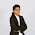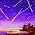## Thursday, 4 January 2018

### Project Expenditure Extraction Query

This query fetches all the expenses incurred on a project,and also captures the debit and credit account transaction wise that will help in reconcilation of data with GL.

SELECT ei.expenditure_id, p.project_id, p.segment1 project_number,p.DESCRIPTION,
p.NAME project_name, ei.expenditure_item_id trans_id, t.task_number,
nvl((select nvl(USER_TRANSACTION_SOURCE,'Pre-Approved Batches')
from pa_transaction_sources where TRANSACTION_SOURCE=ei.transaction_source),'Pre-Approved Batches') transaction_source,
ei.expenditure_type,
(SELECT expenditure_category
FROM pa_expenditure_types
WHERE expenditure_type = ei.expenditure_type) expenditure_category,
ei.expenditure_item_date, vendor_name supp_name,
ap.segment1 supp_numer,
CASE
WHEN ei.transaction_source = 'AP INVOICE'
THEN (SELECT vendor_site_code
FROM ap_supplier_sites_all
WHERE vendor_site_id =
(SELECT vendor_site_id
FROM ap_invoices_all
WHERE invoice_id =
pcla.system_reference2))
WHEN ei.transaction_source = 'PO RECEIPT'
THEN (SELECT vendor_site_code
FROM ap_supplier_sites_all
WHERE vendor_site_id =
(SELECT vendor_site_id
pcla.system_reference2))
ELSE NULL
END vendor_site_code,
ei.quantity, ei.raw_cost_rate,
(SELECT SEGMENT4--concatenated_segments
FROM gl_code_combinations_kfv
WHERE code_combination_id = dr_code_combination_id) d_account,
ei.projfunc_currency_code, ei.project_currency_code,
ei.denom_currency_code trans_cur, ei.acct_raw_cost fun_raw_cost,
ei.project_raw_cost, ei.denom_raw_cost tran_raw_cost,
DECODE
(ei.unit_of_measure,
NULL, pa_utils4.get_unit_of_measure (ei.expenditure_type),
ei.unit_of_measure
) unit_of_measure,                         --ei.unit_of_measure uom,
pa_utils4.get_unit_of_measure_m (ei.unit_of_measure,
ei.expenditure_type
) unit_of_measure_m,
(SELECT expenditure_comment
WHERE expenditure_item_id =
ei.expenditure_item_id)
expenditure_comment,
x.expenditure_group batch_name,
x.expenditure_status_code expend_status_code, ei.cost_distributed_flag,
CASE
WHEN ei.transaction_source = 'AP INVOICE'
THEN (SELECT h.segment1
FROM po_headers_all h, po_lines_all l
AND l.po_line_id = ei.po_line_id)
WHEN ei.transaction_source = 'PO RECEIPT'
THEN (SELECT segment1
WHERE po_header_id = pcla.system_reference2)
ELSE NULL
END po_number,
CASE
WHEN ei.transaction_source = 'AP INVOICE'
THEN NULL
WHEN ei.transaction_source = 'PO RECEIPT'
THEN (SELECT receipt_num
WHERE shipment_header_id IN (
FROM rcv_transactions
pcla.system_reference2
and  TRANSACTION_ID=pcla.system_reference4
))
ELSE NULL
END receipt_number,
(SELECT invoice_num
FROM ap_invoices_all
WHERE invoice_id = pcla.system_reference2) invoice_number,
o1.NAME expenditure_organization_name,
ei.expenditure_item_date gl_date,
(SELECT SEGMENT4--concatenated_segments
FROM gl_code_combinations_kfv
WHERE code_combination_id = cr_code_combination_id) offset_account,
pcla.system_reference2, dr_code_combination_id, cr_code_combination_id,
(SELECT hp.party_name
FROM hz_parties hp, hz_cust_accounts hca
WHERE hp.party_id = hca.party_id
AND hca.status = 'A'
and hca.org_id=p.org_id
AND hca.cust_account_id = ei.attribute1) attribute1,
(SELECT  hcsu.LOCATION
FROM hz_cust_acct_sites_all hcas,
hz_cust_site_uses_all hcsu
WHERE hcas.cust_account_id = ei.attribute1
AND hcas.cust_acct_site_id = hcsu.cust_acct_site_id
AND hcas.cust_acct_site_id = ei.attribute2
and hcas.org_id=p.org_id
and hcsu.site_use_code='BILL_TO') attribute2, ei.po_line_id,
ei.orig_transaction_reference
FROM pa_projects_all p,
pa_expenditure_items_all ei,
pa_expenditures_all x,
pa_project_types_all pt,
pa_transaction_sources tr,
hr_all_organization_units_tl o1,
ap_suppliers ap,
pa_cost_distribution_lines_all pcla
WHERE  t.project_id = p.project_id
AND ei.project_id = p.project_id
AND p.project_type = pt.project_type
AND p.org_id = pt.org_id
AND ei.expenditure_id = x.expenditure_id
AND ap.vendor_id(+) = ei.vendor_id
AND t.project_id = pcla.project_id(+)
AND p.org_id = pcla.org_id(+)
AND ei.expenditure_item_id = pcla.expenditure_item_id(+)
AND NVL (ei.override_to_organization_id, x.incurred_by_organization_id) =
o1.organization_id
AND ei.transaction_source = tr.transaction_source(+)
AND ei.project_id IN (
SELECT prp.project_id
FROM pa_project_players prp, pa_project_role_types_b pprt
WHERE p.project_id = prp.project_id
AND t.project_id = prp.project_id
AND prp.project_role_type = pprt.project_role_type
AND prp.project_role_type IN ('PROJECT MANAGER', 1000)
AND prp.person_id = :p_person_id
AND nvl(prp.end_date_active,SYSDATE) >=SYSDATE)
AND ei.expenditure_item_date BETWEEN NVL (:form_item_date,
ei.expenditure_item_date
)
AND NVL (:to_item_date,
ei.expenditure_item_date
)
AND ei.project_id = :p_project_id

1.Nice blog about punchout XML, it's being great to read this.
CXML Punchout

2.Thanks for sharing article about CXML Punchout
CXML Punchout

3.Thanks for sharing this blog. The content is beneficial and useful. Very informative post.
finance write for us